# Selina solutions for Concise Physics Class 10 ICSE chapter 5 - Refraction through a Lens [Latest edition]

#### Chapters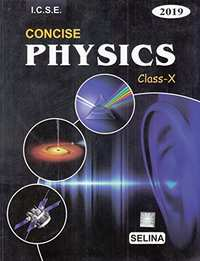## Chapter 5: Refraction through a Lens

Exercise 5 (A)OthersExercise 5 (B)Exercise 5 (C)Exercise 5 (D)
Exercise 5 (A) [Pages 109 - 110]

### Selina solutions for Concise Physics Class 10 ICSE Chapter 5 Refraction through a Lens Exercise 5 (A) [Pages 109 - 110]

Exercise 5 (A) | Q 1 | Page 109

What is a lens?

Exercise 5 (A) | Q 2 | Page 109

Name the different kinds of lenses. Draw diagrams to illustrate them.

Exercise 5 (A) | Q 3 | Page 109

State two differences between a convex and a concave lens in their (a) appearence, and (b) action on the incident light.

Exercise 5 (A) | Q 4.1 | Page 109

Which lens is converging: An equiconcave lens or an equiconvex lens.

Exercise 5 (A) | Q 4.2 | Page 109

Which lens is converging:

A concavo-convex lens or a convexo-concave lens?

Exercise 5 (A) | Q 5 | Page 109

Out of the two lenses, one concave and the other convex, state which one will show the divergent action on a light beam.

Exercise 5 (A) | Q 6 | Page 109

Show by a diagram the refraction of two light rays incident parallel to the principal axis on a convex lens by treating it as a combination of a glass block and two triangular glass prisms.

Exercise 5 (A) | Q 7 | Page 109

Show by a diagram, the refraction of two light rays incident parallel to the principal axis on a concave lens by treating it as a combination of a glass block and two triangular glass prisms.

Exercise 5 (A) | Q 8 | Page 109

How does the action of a convex lens differ from that of a concave lens on a parallel beam of light incident on them? Draw diagram to illustrate your answer.

Exercise 5 (A) | Q 9 | Page 109

Define the term principal axis of a lens.

Exercise 5 (A) | Q 10 | Page 109

Explain optical centre of a lens with the help of proper diagram(s)

Exercise 5 (A) | Q 11 | Page 109

A ray of light incident at a point on the principal axis of a convex lens passes undeviated through the lens.
(a) What special name is given to this point on the principal axis?
(b) Draw a labelled diagram to support your answer in part (a)

Exercise 5 (A) | Q 12 | Page 109

State the condition when a lens is called an equi-convex or equi-concave

Exercise 5 (A) | Q 13 | Page 109

Define the term principal foci of a convex lens and illustrate your answer with the aid of proper diagrams.

Exercise 5 (A) | Q 14 | Page 109

Define the term principal foci of a concave lens and show them with the help of proper diagrams.

Exercise 5 (A) | Q 15 | Page 109

Draw a diagram to represent the second focus of a concave lens.

Exercise 5 (A) | Q 16 | Page 109

Draw a diagram to represent the second focus of a convex lens.

Exercise 5 (A) | Q 17 | Page 109

A ray of light, after refraction through a concave lens emerges parallel to the principal axis. (a) draw a ray diagram to show the incident ray and its corresponding emergent ray. (b) The incident ray when produces meets the principal axis at a point. Name the point.

Exercise 5 (A) | Q 18 | Page 109

A ray of light after refraction through a convex lens emerges parallel to principal axis. (a) Draw a ray diagram to show it. (b) The incident ray passes through a point on the principal axis. Name the point

Exercise 5 (A) | Q 19 | Page 109

A beam of light incident on a convex lens parallel to its principal axis converges at a point on the principal axis. Name the point. Draw a ray diagram to show it.

Exercise 5 (A) | Q 20 | Page 109

A beam of light incident on a thin concave lens parallel to its principal axis diverges and appears to come from a point on the principal axis. Name the point. Draw a ray diagram to show it.

Exercise 5 (A) | Q 21 | Page 109

Define the term focal length of a lens.

Exercise 5 (A) | Q 22 | Page 109

What do you mean by focal plane of a lens?

Exercise 5 (A) | Q 23.1 | Page 109

State the condition for the following a lens has both its focal lengths equal .

Q 23.2 | Page 109

State the condition for the following  a ray passes undeviated through the lens .

Exercise 5 (A) | Q 24 | Page 109

A parallel oblique beam of light falls on a (i) convex lens, (ii) concave lens. Draw a diagram in each case to show the refraction of light through the lens.

Exercise 5 (A) | Q 25.1 | Page 109

The diagram alongside shows a lens as a combination of a glass block and two prisms.  Name the lens formed by the combination.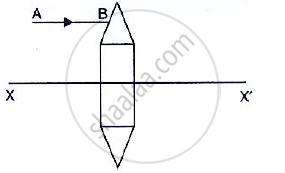Exercise 5 (A) | Q 25.2 | Page 109

The diagram alongside shows a lens as a combination of a glass block and two prisms.

what is the rays the line XX’ called?Exercise 5 (A) | Q 25.3 | Page 109

The diagram alongside shows a lens as a combination of a glass block and two prisms.

Complete the ray diagram and show the path of the incident ray AB after passing through the lensExercise 5 (A) | Q 25.4 | Page 109

The diagram alongside shows a lens as a combination of a glass block and two prisms.

The final emergent ray will either meet XX’ at a point or appear to come from a point on XX’. Label the point as F. What is this point called?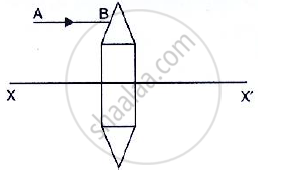Exercise 5 (A) | Q 26 | Page 109

The diagram alongside shows a lens as a combination of a glass block and two prisms.
(i) Name the lens formed by the combination.
(ii) what is the line XX’ called?
(iii) Complete the path of the incident ray AB after passing through the lens.
(iv) The final emergent ray either meets XX’ at a point or appears to come from a point on XX’ Label it as F. what is this point called?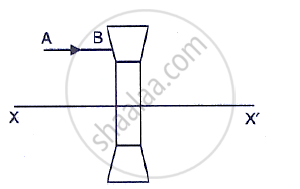Exercise 5 (A) | Q 27 | Page 109

In following figure ,, F1 and F2 are the positions of the two foci of the thin lenses shown in diagram (a) and (b) draw accurately the path taken by the light ray AB after it emerges from the lens in each diagram (a) and (b).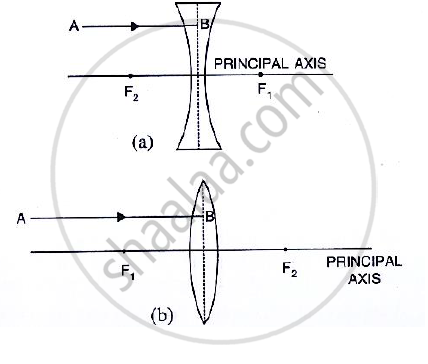Exercise 5 (A) | Q 28 | Page 109

In following figure, F1 and F2 are the two foci of the thin lenses shown in diagram (a) and (b) and AB is the incident ray. Compete the diagram to show the path of the ray AB after refraction through the lens in each diagram (a) and (b).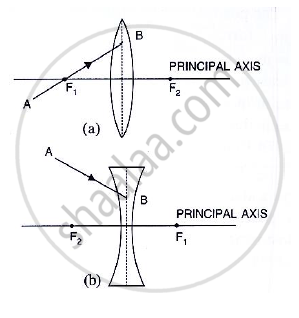Exercise 5 (A) | Q 29.1 | Page 110

Complete the following sentence:

If half part of a convex lens is covered, the focal length ……………… change, but the intensity of image ……………….

Exercise 5 (A) | Q 29.2 | Page 110

Complete the following sentence:

A convex lens is placed in water. Its focal length will ……………………….

Exercise 5 (A) | Q 29.3 | Page 110

Complete the following sentence:

The focal length of a then convex lens is …………….. than that of a thick convex lens.

Exercise 5 (A) [Page 110]

### Selina solutions for Concise Physics Class 10 ICSE Chapter 5 Refraction through a Lens Exercise 5 (A) [Page 110]

Exercise 5 (A) | Q 1 | Page 110

A ray of light after refraction through a lens emerges parallel to the principal axis of the lens. The incident ray either passes through :

• its optical centre

• its first focus

• its second focus

• its centre of curvature of the first surface

Exercise 5 (A) | Q 2 | Page 110

A ray of light incident on a lens parallel to its principal axis, after refraction passes through or appears to come from:

• Its first focus

• Its optical entre

• Its second focus

• The centre of curvature of its second surface

Exercise 5 (B) [Pages 119 - 121]

### Selina solutions for Concise Physics Class 10 ICSE Chapter 5 Refraction through a Lens Exercise 5 (B) [Pages 119 - 121]

Exercise 5 (B) | Q 1 | Page 119

What are the three principal rays that are drawn to construct the ray diagram for the image formed by a lens? Draw diagrams to support your answer.

Exercise 5 (B) | Q 2 | Page 119

In the diagram below, XX’ represents the principal axis, O the optical centre and F the focus of the lens. Complete the path of rays A and B as they emerge out of the lens.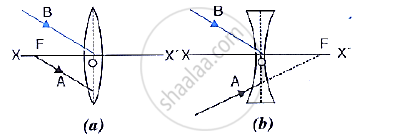Exercise 5 (B) | Q 3 | Page 119

Where must a point source of light be placed in front of a convex lens so as to obtain a parallel beam of light?

Exercise 5 (B) | Q 4 | Page 119

Distinguish between a real and a virtual image.

Exercise 5 (B) | Q 5.1 | Page 119

Study the diagram given below.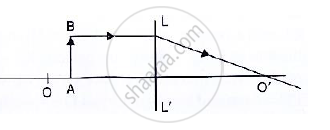Name the lens LL’

Exercise 5 (B) | Q 5.2 | Page 120

Study the following diagram :What are the points O and O’ called?

Exercise 5 (B) | Q 5.3 | Page 120

Study the following  diagram :Complete the diagram to form the image of the object AB.

Exercise 5 (B) | Q 5.4 | Page 120

Study the following diagram :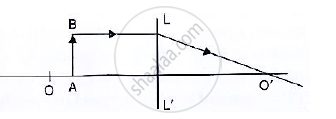state the three characteristics of the image.

Exercise 5 (B) | Q 5.5 | Page 120

Study the following diagram :Name a device in which this action of lens is used.

Exercise 5 (B) | Q 6.1 | Page 120

Study the following diagram :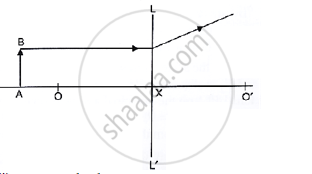Name the lens LL’

Exercise 5 (B) | Q 6.2 | Page 120

Study the diagram below.what are the points O, O’ called?

Exercise 5 (B) | Q 6.3 | Page 120

Study the diagram below.Complete the diagram to form the image of the object AB.

Exercise 5 (B) | Q 6.4 | Page 120

Study the diagram below.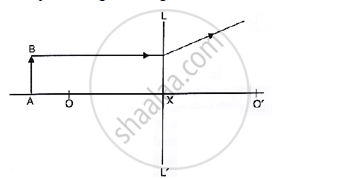State three characteristics of the image

Exercise 5 (B) | Q 7.1 | Page 120

The following diagram shows an object AB and a converging lens L with foci F1 and F2.
Draw two rays from the object and complete the diagram to locate the position of the image. Mark the image CD. Clearly mark on the diagram the position of the eye from where the image can be viewed.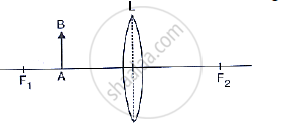Exercise 5 (B) | Q 7.2 | Page 120

The following diagram shows an object AB and a converging lens L with foci F1 and F2 State three characteristics of the image in relation to the object.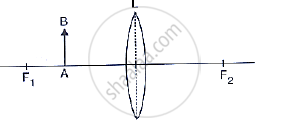Exercise 5 (B) | Q 8.1 | Page 120

The diagram given below shows the position of an object OA in relation to a converging lens whose foci are at F1 and F2.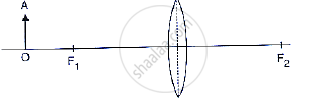draw two rays to locate the position of the image.

Exercise 5 (B) | Q 8.2 | Page 120

The diagram given below shows the position of an object OA in relation to a converging lens whose foci are at F1 and F2.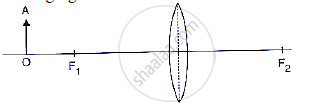State the position of image with reference to the lens.

Exercise 5 (B) | Q 8.3 | Page 120

The diagram given below shows the position of an object OA in relation to a converging lens whose foci are at F1 and F2.Describe three characteristic of the image.

Exercise 5 (B) | Q 8.4 | Page 120

The diagram given below shows the position of an object OA in relation to a converging lens whose foci are at F1 and F2.Describe how the distance of the image from the lens and the size of the image change as the object is moved towards F1.

Exercise 5 (B) | Q 9.1 | Page 120

A converging lens forms the image of an object placed in front of it beyond 2F2 of the lens.

Where is the object placed?

Exercise 5 (B) | Q 9.2 | Page 120

A converging lens forms the image of an object placed in front of it, beyond 2F2 of the lens.

Draw a ray diagram to show the formation of image.

Exercise 5 (B) | Q 9.3 | Page 120

A converging lens forms the image of an object placed in front of it, beyond 2F2 of the lens.

State three characteristics of the image.

Exercise 5 (B) | Q 10.1 | Page 120

A convex lens forms an image of an object equal to the size of the object.Where is the object placed in front of the lens?

Exercise 5 (B) | Q 10.2 | Page 120

A convex lens forms an image of an object equal to the size of the object.draw a diagram to illustrate it.

Exercise 5 (B) | Q 10.3 | Page 120

A convex lens forms an image of an object equal to the size of the object.state two more characteristics of the image.

Exercise 5 (B) | Q 11.1 | Page 120

A lens forms an erect, magnified and virtual image of an object. name the type of lens.

Exercise 5 (B) | Q 11.2 | Page 120

A lens forms an erect, magnified and virtual image of an object.Where is the object placed in relation to the lens?

Exercise 5 (B) | Q 11.3 | Page 120

A lens forms an erect, magnified and virtual image of an object.Draw a ray diagram to show the formation of image.

Exercise 5 (B) | Q 11.4 | Page 120

A lens forms an erect, magnified and virtual image of an object. name the device which uses this principle.

Exercise 5 (B) | Q 12.1 | Page 120

A lens always forms an image between the object and the lens.

name the lens.

Exercise 5 (B) | Q 12.2 | Page 120

A lens always forms an image between the object and the lens.

A lens always forms an image between the object and the lens.

Exercise 5 (B) | Q 12.3 | Page 120

A lens always forms an image between the object and the lens.

state three characteristics of the image.

Exercise 5 (B) | Q 13 | Page 120

Classify as real or virtual, the image of a candle flame formed on a screen by a convex lens. Draw a ray diagram to illustrate how the image is formed.

Exercise 5 (B) | Q 14 | Page 120

Show by a ray diagram that a diverging lens cannot form a real image of an object placed anywhere on its principal axis.

Exercise 5 (B) | Q 15 | Page 120

Draw a ray diagram to show how a converging lens can form a real and enlarged image of an object.

Exercise 5 (B) | Q 16 | Page 120

A lens forms an upright and diminished image of an object irrespective of its position. What kind of lens is this?

Exercise 5 (B) | Q 17 | Page 120

Draw a ray diagram to show how a converging lens is used as a magnifying glass to observe a small object. Mark on your diagram the foci of the lens and the position of the eye.

Exercise 5 (B) | Q 18 | Page 120

Draw a ray diagram to show how a converging lens can form an image of the sun. Hence give a reason for the term ‘burning glass’ for a converging lens used in this manner.

Exercise 5 (B) | Q 19.1 | Page 120

A lens forms an inverted image of an object.  what kind of lens is this?

Exercise 5 (B) | Q 19.2 | Page 120

A lens forms an inverted image of an object what is the nature of the image real or virtual?

Exercise 5 (B) | Q 20.1 | Page 121

A lens forms an upright and magnified image of an object  name the lens

Exercise 5 (B) | Q 20.2 | Page 121

A lens forms an upright and magnified image of an object State whether the image is real or virtual

Exercise 5 (B) | Q 21 | Page 121

a) name the lens which always forms an erect and virtual image.
(b) state whether the image in part (a) is magnified or diminished.

Exercise 5 (B) | Q 22 | Page 121

Can a concave lens form an image of size two times that of the object? Give reason?

Exercise 5 (B) | Q 23 | Page 121

Give two characteristic of the image formed by a concave lens.

Exercise 5 (B) | Q 24 | Page 121

Give two characteristic of the virtual image formed by a convex lens.

Exercise 5 (B) | Q 25.1 | Page 121

In the following cases, where must an object be placed in front of a convex lens so that the image formed is at infinity.

Exercise 5 (B) | Q 25.2 | Page 121

In the following cases, where must an object be placed in front of a convex lens so that the image formed is of same size as the object .

Exercise 5 (B) | Q 25.3 | Page 121

In the following cases, where must an object be placed in front of a convex lens so that the image formed is inverted and enlarged .

Exercise 5 (B) | Q 25.4 | Page 121

In the following cases, where must an object be placed in front of a convex lens so that the image formed is upright and enlarged image?

Exercise 5 (B) | Q 26 | Page 121

Complete the following table:

 Type of lens Position of object Nature of image Size of image Convex Between optic centre and focus Convex At focus Concave At infinity Concave At any distance
Exercise 5 (B) | Q 27 | Page 121

State the changes in the position, size and nature of the image of an object when brought from infinity up to a convex lens. Illustrate your answer by drawing ray diagrams.

Exercise 5 (B) | Q 28 | Page 121

State the changes in the position, size and nature of the image of an object when brought from infinity up to a concave lens. Illustrate your answer by drawing ray diagrams.

Exercise 5 (B) | Q 29.1 | Page 121

Complete the following sentence:

An object is placed at a distance of more than 40 cm from a convex lens of focal length 20 cm. The image formed is real, inverted and ……….

Exercise 5 (B) | Q 29.2 | Page 121

Complete the following sentence:

an object is placed at a distance 2f from a convex lens of focal length f. The size of image formed is ………….. that of the object.

Exercise 5 (B) | Q 29.3 | Page 121

Complete the following sentence:

an object is placed at a distance 5 cm from a convex lens of focal length 10 cm. The image formed is virtual, upright and …………….

Exercise 5 (B) | Q 30.1 | Page 121

State whether the following statement are ‘true’ or ‘false’ by writing T/F against them.

A convex lens has a divergent action and a concave lens has a convergent action.

• True

• False

Exercise 5 (B) | Q 30.2 | Page 121

State whether the following statement are ‘true’ or ‘false’ by writing T/F against them.

A concave lens, if kept at a proper distance from an object, can form its real image.

• True

• False

Exercise 5 (B) | Q 30.3 | Page 121

State whether the following statements\ are ‘true’ or ‘false’ by writing T/F against them.

A ray of light incident parallel to the principal axis of a lens, passes undeviated after refraction.

• True

• False

Exercise 5 (B) | Q 30.4 | Page 121

State whether the following statement are ‘true’ or ‘false’ by writing T/F against them.

A ray of light incident at the optical centre of lens, passes undeviated after refraction

• True

• False

Exercise 5 (B) | Q 30.5 | Page 121

State whether the following statement are ‘true’ or ‘false’ by writing T/F against them.

A concave lens forms a magnified or diminished image depending on the distance of object from it.

• True

• False

Exercise 5 (B) [Page 121]

### Selina solutions for Concise Physics Class 10 ICSE Chapter 5 Refraction through a Lens Exercise 5 (B) [Page 121]

Exercise 5 (B) | Q 1 | Page 121

For an object placed at distance 20 cm in front of a convex lens, the image is at distance 20 cm behind the lens. The focal length of convex lens is:

• 20 cm

• 10 cm

• 15 cm

• 40 cm

Exercise 5 (B) | Q 2 | Page 121

For the object placed between optical centre and focus of a convex lens, the image is:

• Real and enlarged

• Real and diminished

• Virtual and enlarged

• Virtual and diminished.

Exercise 5 (B) | Q 3 | Page 121

A concave lens forms the image of an object which is:

• Virtual, inverted and diminished

• Virtual, upright and diminished

• Virtual, inverted and enlarged

• Virtual, upright and enlarged

Exercise 5 (C) [Page 126]

### Selina solutions for Concise Physics Class 10 ICSE Chapter 5 Refraction through a Lens Exercise 5 (C) [Page 126]

Exercise 5 (C) | Q 1 | Page 126

State the sign convention to measure the distances for a lens.

Exercise 5 (C) | Q 2 | Page 126

The focal length of a lens is (i) positive, (ii) negative.

In each case, state the kind of lens.

Exercise 5 (C) | Q 3 | Page 126

Write the lens formula explaining the meaning of the symbols used.

Exercise 5 (C) | Q 4 | Page 126

What do you understand by the term magnification? Write expression for it for a lens, explaining the meaning of the symbols used.

Exercise 5 (C) | Q 5 | Page 126

What information about the nature of image (i) real or virtual, (ii) erect or inverted, do you get from the sign of magnification + or - ?

Exercise 5 (C) | Q 6 | Page 126

Define the term power of a lens. In what unit is it expressed?

Exercise 5 (C) | Q 7 | Page 126

How is the power of a lens related to its focal length?

Exercise 5 (C) | Q 8 | Page 126

How does the power of a lens change is its focal length is doubled?

Exercise 5 (C) | Q 9 | Page 126

How is the sign (+ Or -) of power of a lens related to its divergent or convergent action?

Exercise 5 (C) | Q 10 | Page 126

The power of a lens is negative. State whether it is convex or concave?

Exercise 5 (C) | Q 11 | Page 126

Which lens has more power: a thick lens or a thin lens?

Q 12

Describe how you would determine the focal length of a converging lens, using a plane mirror and one pin. Draw a ray diagram to illustrate your answer.

Q 13.1

State two applications of a convex lens.

Q 13.2

State two applications  a concave lens .

Q 14

How will you differentiate between a convex and a concave lens by looking at (i) a distant object, (ii) a printed page?

Exercise 5 (B) [Page 126]

### Selina solutions for Concise Physics Class 10 ICSE Chapter 5 Refraction through a Lens Exercise 5 (B) [Page 126]

Exercise 5 (B) | Q 1 | Page 126

If the magnification produced by a lens is - 0.5, the correct statement is :

• The lens is concave

• The image is virtual

• The image is magnified

• The images is real and diminished formed by a convex

Exercise 5 (B) | Q 2 | Page 126

The correct lens formula is

• 1/"u" + 1/"v" = 1/"f"

• 1/"u" - 1/"v" = 1/"f"

• 1/"v" - 1/"u" = 1/"f"

• f = ("u + v")/"uv"

Exercise 5 (B) | Q 3 | Page 126

On reducing the focal length of a lens, its power :

• Decreases

• Increases

• Does not change

• First increases then decreases.

Exercise 5 (B) | Q 4 | Page 126

The power of a lens is + 1.0 D is :

• convex of focal length 1.0 cm

• convex of focal length 1.0 m

• concave of focal length 1.0 cm

• concave of focal length 1.0 m

Exercise 5 (C) [Pages 126 - 127]

### Selina solutions for Concise Physics Class 10 ICSE Chapter 5 Refraction through a Lens Exercise 5 (C) [Pages 126 - 127]

Exercise 5 (C) | Q 1 | Page 126

At what position a candle of length 3 cm be placed in front of a convex lens so that its image of length 6 cm be obtained on a screen placed at distance 30 cm behind the lens?

What is the focal length of lens in part (a)?

Exercise 5 (C) | Q 2 | Page 127

A concave lens forms the image of an object kept at a distance 20 cm in front of it, at a distance 10 cm on the side of the object.

What is the nature of the image?

Find the focal length of the lens.

Exercise 5 (C) | Q 3 | Page 127

The focal length of a convex lens is 25 cm. At what distance from the optical centre of the lens an object be placed to obtain a virtual image of twice the size?

Exercise 5 (C) | Q 4 | Page 127

Where should an object be placed in front of a convex lens of focal length 0.12 m to obtain a real image of size three times the size of the object, on the screen?

Exercise 5 (C) | Q 5 | Page 127

An illuminated object lies at a distance 1.0 m from a screen. A convex lens is used to form the image of object on a screen placed at distance 75 cm from the lens . Find: (i) the focal length of lens, and (ii) the magnification.

Exercise 5 (C) | Q 6.1 | Page 127

A lens forms the image of an object placed at a distance 15 cm from it, at a distance 60 cm in front of it. Find :

the focal length,

Exercise 5 (C) | Q 6.2 | Page 127

A lens forms the image of an object placed at a distance 15 cm from it, at a distance 60 cm in front of it. Find :  the magnification.

Exercise 5 (C) | Q 6.3 | Page 127

A lens forms the image of an object placed at a distance 15 cm from it, at a distance 60 cm in front of it. Find :  the nature of image.

Exercise 5 (C) | Q 7.1 | Page 127

A lens forms the image of an object placed at a distance of 45 cm from it on a screen placed at a distance 90 cm on other side of it. Find the name the kind of lens.

Exercise 5 (C) | Q 7.2 | Page 127

A lens forms the image of an object placed at a distance of 45 cm from it on a screen placed at a distance 90 cm on other side of it. find: (i) the focal length of lens, (ii) the magnification of image.

Exercise 5 (C) | Q 8.1 | Page 127

An object is placed at a distance of 20 cm in front of a concave lens of focal length 20 cm. find : the position of image.

Exercise 5 (C) | Q 8.2 | Page 127

An object is placed at a distance of 20 cm in front of a concave lens of focal length 20 cm. find: the magnification of image.

Exercise 5 (C) | Q 9.1 | Page 127

A convex lens forms an inverted image of size same as that of the object which is placed at a distance 60 cm in front of the lens. Find: The position of image

Exercise 5 (C) | Q 9.2 | Page 127

A convex lens forms an inverted image of size same as that of the object which is placed at a distance 60 cm in front of the lens. Find : The focal length of the lens

Exercise 5 (C) | Q 10.1 | Page 127

A concave lens forms an erect image of 1/3rdsize of the object which is placed at a distance 30 cm in front of the lens. Find : The position of image

Exercise 5 (C) | Q 10.2 | Page 127

A concave lens forms an erect image of 1/3rdsize of the object which is placed at a distance 30 cm in front of the lens. Find: The focal length of the lens.

Exercise 5 (C) | Q 11 | Page 127

The power of a lens is +2.0 D. Find its focal length and state what kind of lens it is?

Exercise 5 (C) | Q 12 | Page 127

Express the power (with sign) of a concave lens of focal length 20 cm.

Exercise 5 (C) | Q 13 | Page 127

The focal length of a convex lens is 25 cm. Express its power with sign.

Exercise 5 (C) | Q 14 | Page 127

The power of a lens is -2.0 D. Find its focal length and its kind.

Exercise 5 (C) | Q 15 | Page 127

The magnification by a lens is -3. Name the lens and state how are u and v related?

Exercise 5 (C) | Q 16 | Page 127

The magnification by a lens is +0.5. Name the lens and state how are u and v related?

Exercise 5 (C) | Q 17 | Page 127

A concave lens is a focal length 30 cm. Find the position and magnification (m) of image for an object placed in front of it at distance 30 cm.State whether the image is real on virtual?

Exercise 5 (C) | Q 18 | Page 127

Find the position and magnification of the image of an object placed at distance of 8.0 cm in front of a convex lens of focal length 10.0 cm. Is the image erect or inverted?

Exercise 5 (D) [Pages 131 - 132]

### Selina solutions for Concise Physics Class 10 ICSE Chapter 5 Refraction through a Lens Exercise 5 (D) [Pages 131 - 132]

Exercise 5 (D) | Q 1 | Page 131

What is magnifying glass? State its two uses.

Exercise 5 (D) | Q 2 | Page 131

Draw a neat labelled ray diagram to show the formation of image by a magnifying glass. State three characteristics of the image.

Exercise 5 (D) | Q 3 | Page 131

Where is the object placed in reference to the principal focus of a magnifying glass, so as to see its enlarged image? Where is the image obtained?

Exercise 5 (D) | Q 4 | Page 131

Define magnifying power of a simple microscope. How can it be increased?

Exercise 5 (D) | Q 5 | Page 131

State two applications each of a convex lens and concave lens.

Exercise 5 (D) | Q 6 | Page 131

Describe in brief how would you determine the approximate focal length of a convex lens.

Exercise 5 (D) | Q 7 | Page 131

The following  diagram shows the experimental set up for the determination of focal length of a lens using a plane mirror.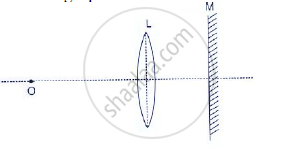(i) draw two rays from the point O of the object to show the formation of image I at O itself.
(ii) What is the size of the image I?
(iii)State two more characteristics of the image I.
(iv) Name the distance of the objects O from the optical centre of the lens.
(v) To what point will the rays return if the mirror is moved away from the lens by a distance equal to the focal length of the lens ?

Exercise 5 (D) | Q 8 | Page 132

Describe how you would determine the focal length of a converging lens, using plane mirror and one pin. Draw a ray diagram to illustrate your answer.

Exercise 5 (D) | Q 9.1 | Page 132

How will you differentiate between a convex and a concave lens by looking at  a distant object.

Exercise 5 (D) | Q 9.2 | Page 132

How will you differentiate between a convex and a concave lens by looking at a printed page?

Exercise 5 (D) [Page 132]

### Selina solutions for Concise Physics Class 10 ICSE Chapter 5 Refraction through a Lens Exercise 5 (D) [Page 132]

Exercise 5 (D) | Q 1 | Page 132

A magnifying glass forms :

• A real and diminished image

• A real and magnified image

• A virtual and magnified image

• A virtual and diminished image

Exercise 5 (D) | Q 2 | Page 132

The maximum magnifying power of a convex lens of focal length 5 cm can be:

• 25

• 10

• 1

• 6

## Chapter 5: Refraction through a Lens

Exercise 5 (A)OthersExercise 5 (B)Exercise 5 (C)Exercise 5 (D)## Selina solutions for Concise Physics Class 10 ICSE chapter 5 - Refraction through a Lens

Selina solutions for Concise Physics Class 10 ICSE chapter 5 (Refraction through a Lens) include all questions with solution and detail explanation. This will clear students doubts about any question and improve application skills while preparing for board exams. The detailed, step-by-step solutions will help you understand the concepts better and clear your confusions, if any. Shaalaa.com has the CISCE Concise Physics Class 10 ICSE solutions in a manner that help students grasp basic concepts better and faster.

Further, we at Shaalaa.com provide such solutions so that students can prepare for written exams. Selina textbook solutions can be a core help for self-study and acts as a perfect self-help guidance for students.

Concepts covered in Concise Physics Class 10 ICSE chapter 5 Refraction through a Lens are Magnification Due to Spherical Lenses, Lens, Action of a Lens as a Set of Prisms, Spherical Lens, Refraction of Light Through the Equiconvex Lens and Equiconcave Lens, Guideline for Image Formation Due to Refraction Through a Convex and Concave Lens, Formation of Image by Reflection: Real and Virtual Image, Images Formed by Sperical Lenses, Concave Lens, Images Formed Due to Refraction Through a Concave Lens, Convex Lens, Images Formed Due to Refraction Through a Convex Lens, Differentiation Between Concave and Convex Lens, Sign Convention for Spherical Lenses, Lens Formula, Power of a Lens, Magnifying Glass Or Simple Microscope, Experimental Determination of Focal Length of Convex Lens.

Using Selina Class 10 solutions Refraction through a Lens exercise by students are an easy way to prepare for the exams, as they involve solutions arranged chapter-wise also page wise. The questions involved in Selina Solutions are important questions that can be asked in the final exam. Maximum students of CISCE Class 10 prefer Selina Textbook Solutions to score more in exam.

Get the free view of chapter 5 Refraction through a Lens Class 10 extra questions for Concise Physics Class 10 ICSE and can use Shaalaa.com to keep it handy for your exam preparation